# Power and Efficiency

## Power

Power is the rate of using energy.

Power is measured in Watts (W). 1W is 1J of energy being used per second.A 100W light bulb will use more electrical energy than a 60W bulb if they are both on for the same length of time.

You need to remember the equation for power for your exam:

## P = E ÷ t

__P __= power (W)

E = work done or energy transferred (J)

t = time (s)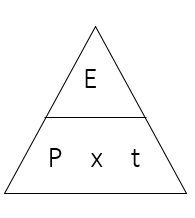There are also two other equations for power that you need to memorise which will be covered in more detail in the electricity lessons.

Power = Current x Voltage

## P = IV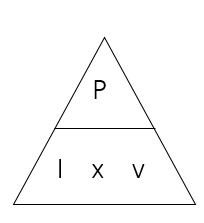And:

Power = Current2__ x Resistance__

## Converting Units

It is important to be able to convert units as power is often given in kilowatts (kW).

1 kW = 1000W

To change W into kW, divide by 1000.

To change kW into W, multiply by 1000.

Worked ExampleA weightlifter raises a bar from the floor to above his head. To lift the bar, the weightlifter does 5kJ of work in 2.5s.

Calculate the power he produced:

1. Power = energy ÷ time
2. 5kJ = 5000J
3. Power = 5000 ÷ 2.5 = 2000 W

Another weightlifter lifts the same weight in a shorter time. Compare the power of the two weightlifters.

1. Using the same amount of energy in a shorter time would require more power

## Sankey Diagrams

Sankey diagrams were introduced in a previous lesson. They can be used to calculate efficiency.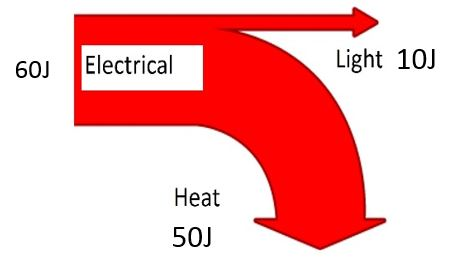For this Sankey diagram, the useful energy output is 10J and the total energy input is 60J. Therefore, the efficiency is 10÷60=0.17 (or 17%)

## Efficiency

All devices waste some energy, usually as heat due to friction between moving parts.

1. For example, a light bulb is designed to produce light, but also produces heat energy which is dissipated into the surroundings.
2. A car engine is designed to produce kinetic energy but also produces heat and sound which is dissipated into the surroundings.

Efficiency is a measurement of how much energy is converted usefully (compared to how much is wasted).

You need to remember the equations for efficiency:

Or

## Efficiency = useful power out ÷ total power in

Efficiency has no units!

It can be written as a number between 0 and 1 or as a %. For example, an efficiency of 0.25 is the same as an efficiency of 25%.

Because some energy is always wasted from every device, efficiency should always be less than 1 or less than 100%. If you get answer that is bigger than 1 or 100%, you have made a mistake somewhere in your calculation!

Worked ExamplesA power drill uses 500J of electricity. Only 400J of kinetic energy was generated by the drill. What is its efficiency?

1. Efficiency = useful energy out ÷ total energy in
2. Efficiency = 400 ÷ 500
3. Efficiency = 0.8 (or 80%)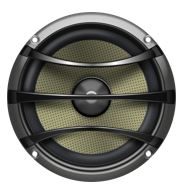A portable speaker coverts 150J of electrical energy into 30J of sound energy. Calculate its efficiency.

1. Efficiency = useful energy out ÷ total energy in
2. Efficiency = 30 ÷ 150
3. Efficiency = 0.2 (or 20%)

## Energy Saving Bulbs

Traditional, filament light bulbs use electricity flowing through a thin piece of wire called a filament. The filament gets hot and glows, producing light. As a lot of heat is also produced, filament bulbs are not very efficient.

Energy saving light bulbs do not use this method and are more efficient. This means that, for the same amount of electrical energy, the energy saving bulb will produce more light than the filament bulb.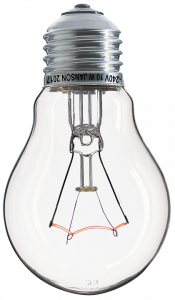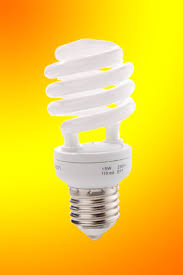Energy saving bulbs are usually more expensive to buy compared to filament bulbs. However, the energy saving bulb has a much longer lifetime than the filament bulb, which will need to be replaced regularly. Because the filament bulb is less efficient, the cost of electricity for the filament bulb will be much higher than the energy saving bulb. So, although the energy efficient bulb has a higher initial cost, it will save money in the long term.

A or B? Which of these devices uses the most energy? A - a 2kW heater used for 4 hours. B - a 3kW fire used for 3 hours.
B
A or B? Which of these devices uses the most energy? A - a 1kW iron used for 1 hours. B - a 2kW kettle used for 20 minutes.
A
An electric motor uses 275J to lift a load in 5s. What is the power of the motor?